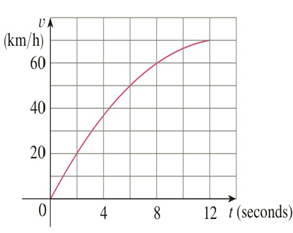Chapter 5.5, Problem 16E

Chapter
Section
Textbook Problem

# The velocity graph of an accelerating car is shown.(a) Use the Midpoint Rule to estimate the average velocity of the car during the first 12 seconds.(b) what time was the instantaneous velocity equal to the average velocity?

To determine

(a)

To estimate:

The average velocity of a car during the first 12 seconds using the midpoint rule.

Explanation

1) Concept:

Use the mean value theorem for integrals and the midpoint rule to estimate the average velocity of the car.

2) Mean Value Theorem for IntegralsandMidpoint rule:

The Mean Value Theorem: If f is continuous on a,b then there exists a number c in a,b such that

fc=fave=1b-aabfx dx

that is,

abfx dx=fcb-a

The midpoint rule:

abfxdxi=1nfxi- x=x fx1-++fxn-

where x=b-an  and  xi-=12xi-1+xi midpoint of xi-1, xi.

3) Calculation:

By using the mean value theorem for integrals:

Here,a=0 and b=12

The average velocity of the car during the first 12 seconds means the average value of v on 0,12.

vave=112-0012vx dx

vave=112012vx dx

Since we can change the variable in the integration,

vave=112012vtdt

Use themidpoint rule.

With n=3, a=0 and b=12 interval width is

t=b-an

Substitute values.

t=12-03

t=4

The three subintervals are 0,4, 4,8, 8,12

To determine

(b)

To find:

The time where the instantaneous velocity equal to the average velocity

### Still sussing out bartleby?

Check out a sample textbook solution.

See a sample solution

#### The Solution to Your Study Problems

Bartleby provides explanations to thousands of textbook problems written by our experts, many with advanced degrees!

Get Started

#### Evaluate the limit. limxe2xe2xln(x+1)

Single Variable Calculus: Early Transcendentals, Volume I

#### In Exercises 6372, evaluate the expression. 68. 1+22

Applied Calculus for the Managerial, Life, and Social Sciences: A Brief Approach

#### In problems 63-73, factor each expression completely. 72.

Mathematical Applications for the Management, Life, and Social Sciences

#### The scalar projection of on is:

Study Guide for Stewart's Multivariable Calculus, 8th

#### True or False: is a rational function.

Study Guide for Stewart's Single Variable Calculus: Early Transcendentals, 8th